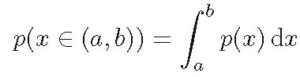# Machine Learning 101 – What is Probability Density?

By now, you probably understand probability as well as probability theory. You also know about the Sum Rule and Product Rule. Then  you also understand Bayes’ theorem from Lesson 9 and  Lesson 10. However, you also need to understand the term Probability Density.

To understand Probability Density, you need to understand the following:

• difference between continuous and discrete variable
• what is a random variable
• probability of a random variable taking on a given value

Let’s start with the first one because we mentioned it when we in Lecture 3 under difference between Classification and Regression.

difference between continuous and discrete variable

A discrete variable is one that can take one finite range of values. For example integers between 1 and 9. In this case they are 1, 2, 3, 4, 5, 6, 7, 8 and 9. Another example is result of a cancer test. It could be one of two values: positive or negative.

On the other hand, a continuous variable can take on infinite range of values. For example, real numbers between 1 and 0. In this case it could be 1, 1.01, 1.004, 1.333, 2.32, …. and so on. The combination is infinite.

You can therefore recall that in classification, we are trying to predict the value of a discreet variable but in regression, we are trying to predict value of a continuous variable.

what is a random variable

A random variable is a variable whose possible values are from the outcome of a random experiment.  For example, in the experiment of choosing a fruit from a box containing oranges and apples. If the outcome of choosing a fruit is F, then F can be either apple(a) or orange (o).

So we can write it as F = a or F = o. Here F is  a random variable, while o or a are values of the random variable.

probability of a random variable taking on a given value

This is kind of self-explanatory.  So using the example of box of fruits,  we can represent the probability of a random variable  as:

P(F=a): this means the probability of the random variable F taking the value a. This is normally written as P(a)

P(F = o): this means the probability of the random variable taking the value o. Written as P(o)

#### Probability Density

The probability density is the probability that a random variable takes on a value between two values.

Now, we can apply this to continuous variables. Consider a continuous random variable x.

Let p(x) be the probability that x takes certain values. This function is called the probability density function. Then the values of p(x) can range from 0 to 1.

Also, x can take on any value from 0 to infinity.

Now, we can try to calculate the probability that the random variable x takes a value between a and b.  The result will be the sum of all the probabilities for values from a to b. We call this the probability density over x.

This sum can be written in integral form as:More formally we say that, if the probability of a random variable x falling in the interval (x,  x + δx) is given by  p(x)δx    for    δx → 0,   then p(x) is the probability density over x.

I you know a bit of calculus, then you will recognize that the probability density is the same as the shaded area in Figure 1 below.

Final Notes

This is the much we’ll taken on Probability Density and Probability Density Function.

Although there are a few other terms but I’ll rather say you don’t worry about them for now. For instance, cumulative distribution function and probability mass function#### kindsonthegenius

Kindson Munonye is currently completing his doctoral program in Software Engineering in Budapest University of Technology and Economics

View all posts by kindsonthegenius →